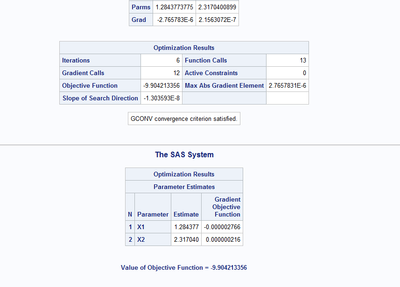## non-linear optimisation BFGS (proc nlpqn)

I am trying to implement a non linear optimisation for a non-hidden multi-state markov model. A similar methodology, if you are interested, can be found in the msm R package (methodology explained in https://cran.r-project.org/web/packages/msm/vignettes/msm-manual.pdf).

the input data are composed by:

TIMELAG NOCC TR1 TR2 TR3 TR4

timelag is a float value indicating the timelag of the transition

nocc is an integer indicating how many times the transition is happening

tr1 is 0/1 depending on whether this transition is 0-0 or not

tr2 is 0/1 depending on whether this transition is 0-1 or not

tr3 is 0/1 depending on whether this transition is 1-0 or not

tr4 is 0/1 depending on whether this transition is 1-1 or not

(I need the 4 transition variables to understand with IML which record to select of the Pmatrix below)

what I want to optimise is basically a piecewise likelihood function, for every record I will have a contribution, then I'll sum all contributions and that will be my likelihood function.

0.3, 0.7

then compose the Qmatrix as:

-0.3 0.3 ,

0.7 -0.7

the create the Pmatrix for each record as:

exp(timelag*qmat11)      exp(timelag*qmat11)*qmat12

exp(timelag*qmat22)*qmat21        exp(timelag*qmat22)

then depending on which type of transition it is, I use as contribution to the likelihood the respective record in the pmatrix, e.g. if 0-0 I select Pmat11.

since this computation has to happen for every record, I thought it might have been much simpler to do that rowwise, so instead of using 2x2 matrixes, I will have a vector with 4 elements as qmatrix for each record, a vector with 4 elements as pmatrix for each record, then sum the product between pmatrix columns and tr1 tr2 tr3 tr4 columns, in order to save only the correct contribution.

``````proc iml;
reset noname;

use mod10;
read all var {WHICHA TIMELAG NOCC} into myds;
read all var {TR1 TR2 TR3 TR4} into trds;
close mod10;

start mylik(x) global(myds,trds);
qmat=shape(-x,nrow(myds),1)||shape(x,nrow(myds),1)||shape(x,nrow(myds),1)||shape(-x,nrow(myds),1);
pmat=exp(myds[,2]#qmat[,1])||exp(myds[,2]#qmat[,1])#qmat[,2]||exp(myds[,2]#qmat[,4])#qmat[,3]||exp(myds[,2]#qmat[,4]);
contrib=pmat[,1]#trds[,1]+pmat[,2]#trds[,2]+pmat[,3]#trds[,3]+pmat[,4]#trds[,4];
loglik=-2#log(sum(contrib#myds));
return(loglik);
finish mylik;

x={0.1,0.8};

opt={0 5 . 3};
call nlpqn(rc,xr,"mylik",x,opt);
quit;``````

I am here not to ask you about the contruction of pmatrix, qmatrix and selection of contrib, this part might be better of course, but it works and I am happy with it.

What I really need help with is the optimisation part.

In order to mimic the methodology of the R paper I am trying to implement BFGS, with CALL NLPQN opt=3.

Basically I can't manage to get SAS to start the optimisation.

Any help on this part? what am I missing?

below some code to mimic the input dataset:

```data mod10;
call streaminit(123);
whicha=0;
do rky=1 to 4;
tr1=0;
tr2=0;
tr3=0;
tr4=0;
if rky=1 then tr1=1;
if rky=2 then tr2=1;
if rky=3 then tr3=1;
if rky=4 then tr4=1;
do rkk=1 to 100;
if rand("Uniform")>.5 then do;
whicha=whicha+1;
timelag=rkk;
nocc=1+round(rand("Uniform")*2,1);
output;
end;
end;
end;
run;

proc iml;
reset noname;

use mod10;
read all var {WHICHA TIMELAG NOCC} into myds;
read all var {TR1 TR2 TR3 TR4} into trds;
close mod10;

print myds trds;

start mylik(x) global(myds,trds);
qmat=shape(-x,nrow(myds),1)||shape(x,nrow(myds),1)||shape(x,nrow(myds),1)||shape(-x,nrow(myds),1);
pmat=exp(myds[,2]#qmat[,1])||exp(myds[,2]#qmat[,1])#qmat[,2]||exp(myds[,2]#qmat[,4])#qmat[,3]||exp(myds[,2]#qmat[,4]);
contrib=pmat[,1]#trds[,1]+pmat[,2]#trds[,2]+pmat[,3]#trds[,3]+pmat[,4]#trds[,4];
loglik=-2#log(sum(contrib#myds));
return(loglik);
finish mylik;

x={0.1,0.8};

opt={0 5 . 3};
call nlpqn(rc,xr,"mylik",x,opt);

quit;```

3 REPLIES 3

## Re: non-linear optimisation BFGS (proc nlpqn)

I'm on vacation until Tuesday, but here are three hints:

1. Double check your objective function. Usually, we compute SUM(LOG(...)) , not LOG(SUM(...)).

2. Look at this blog post: Two ways to compute maximum likelihood estimates in SAS - The DO LoopKsharp
Super User

## Re: non-linear optimisation BFGS (proc nlpqn)

```1)
I think the problem is you are using EXP() funtion for call nlpqn() .
Variable "timelag" has value like >60 is too way big for EXP().
If you make it smaller, you would get convergency result, Like:

timelag=rkk;
-->
timelag=rkk*0.01;

2)
And "loglik=-2#log(sum(contrib#myds));" should be "myds[ ,3]"  ??
```## Re: non-linear optimisation BFGS (proc nlpqn)

Thanks, I fixed this part, rick was right, it was a mistake in the conceptual order of sum and log.

Thanks for your support, iml is not my usual thing, but I'm becoming more and more interested in it with this exercise.

I'm now working on the gradient function, will get back if I still run into issues.
From The DO Loop